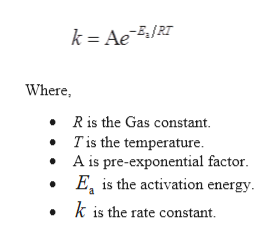# BRIUMKINANDUsing the Arrhenius equation to calculate k at one temperature...DENNISThe rate constant of a certain reaction is known to obey the Arrhenius equation, and to have an activation energy E8.0 kJ/mol. If the rate constant of thisMSreaction is 2.1 x 10at 338.0 °C, what will the rate constant be at 414.0 °C?Round your answer to 2 significant digits.- 1S- 1k = l Mx10X18AExplanationCheck2019 McGraw-Hill Education. All Rights Reserved. Terms of Use Privacy

Question
1 views

If the rate constant of this reaction is 2.1×10^9 add 338 Celsius what will the rate constant be at 114 Celsius. Round to two significant digits.

check_circle

Step 1

Given information:

Activation energy (Ea) = 8.0 kJ/mol

Initial temperature (T1) = 338.0 ̊C

Final temperature (T2) = 414.0 ̊C

Rate constant (k1) = 2.1×109 M-1.s-1

Step 2

Activation energy is the minimum amount of energy required by atoms to overcome the energy barrier. The relation between activation energy and rate of reaction is given by an expression as shown below:help_outlineImage Transcriptionclosek AeRT Where, Ris the Gas constant Tis the temperature A is pre-exponential factor E is the activation energy k is the rate constant fullscreen
Step 3

The activation energy at different rate constant...

### Want to see the full answer?

See Solution

#### Want to see this answer and more?

Solutions are written by subject experts who are available 24/7. Questions are typically answered within 1 hour.*

See Solution
*Response times may vary by subject and question.
Tagged in

### Chemical Kinetics